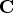Previous Page | Next Page

 Language Reference

## SETDIF Function

compares elements of two matrices

SETDIF( matrix1, matrix2)

The inputs to the SETDIF function are as follows:
matrix1
is a reference matrix. Elements of matrix1 not found in matrix2 are returned in a vector. It can be either numeric or character.

matrix2
is the comparison matrix. Elements of matrix1 not found in matrix2 are returned in a vector. It can be either numeric or character, depending on the type of matrix1.
The SETDIF function returns as a row vector the sorted set (without duplicates) of all element values present in matrix1 but not in matrix2. If the resulting set is empty, the SETDIF function returns a null matrix (with zero rows and zero columns). The argument matrices and result can be either both character or both numeric. For character matrices, the element length of the result is the same as the element length of the matrix1. Shorter elements in the second argument are padded on the right with blanks for comparison purposes.

For example, the following statements produce the matrix, as shown:

```
a={1 2 4 5};
b={3 4};
c=setdif(a,b);
```

```
C             1 row       3 cols    (numeric)

1         2         5
```Previous Page | Next Page | Top of Page